# AP Calculus AB Practice Test 26

### Test Information10 questions20 minutes

Calculator Disallowed

1.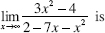2.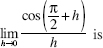3. If, for all x, f (x) = (x - 2)4 (x - 1)3, it follows that the function f has

4. Let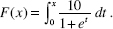Which of the following statements is (are) true?

I. F (0) = 5

II. F(2) < F(6)

III. F is concave upward.

5. If f (x) = 10x and 101.0410.96, which is closest to f (1)?

6. If f is differentiable, we can use the line tangent to f at x = a to approximate values of f near x = a. Suppose this method always underestimates the correct values. If so, then at x = a, the graph of f must be

7. If f (x) = cos x sin 3x, then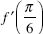is equal to

8.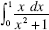is equal to

9. The graph of f is shown below. If f (1) = 0, then f (x) = 0 at x =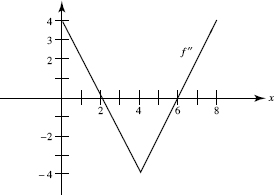10. Use the following table, which shows the values of differentiable functions f and g.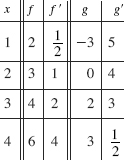If P(x) = g2 (x), then P (3) equals